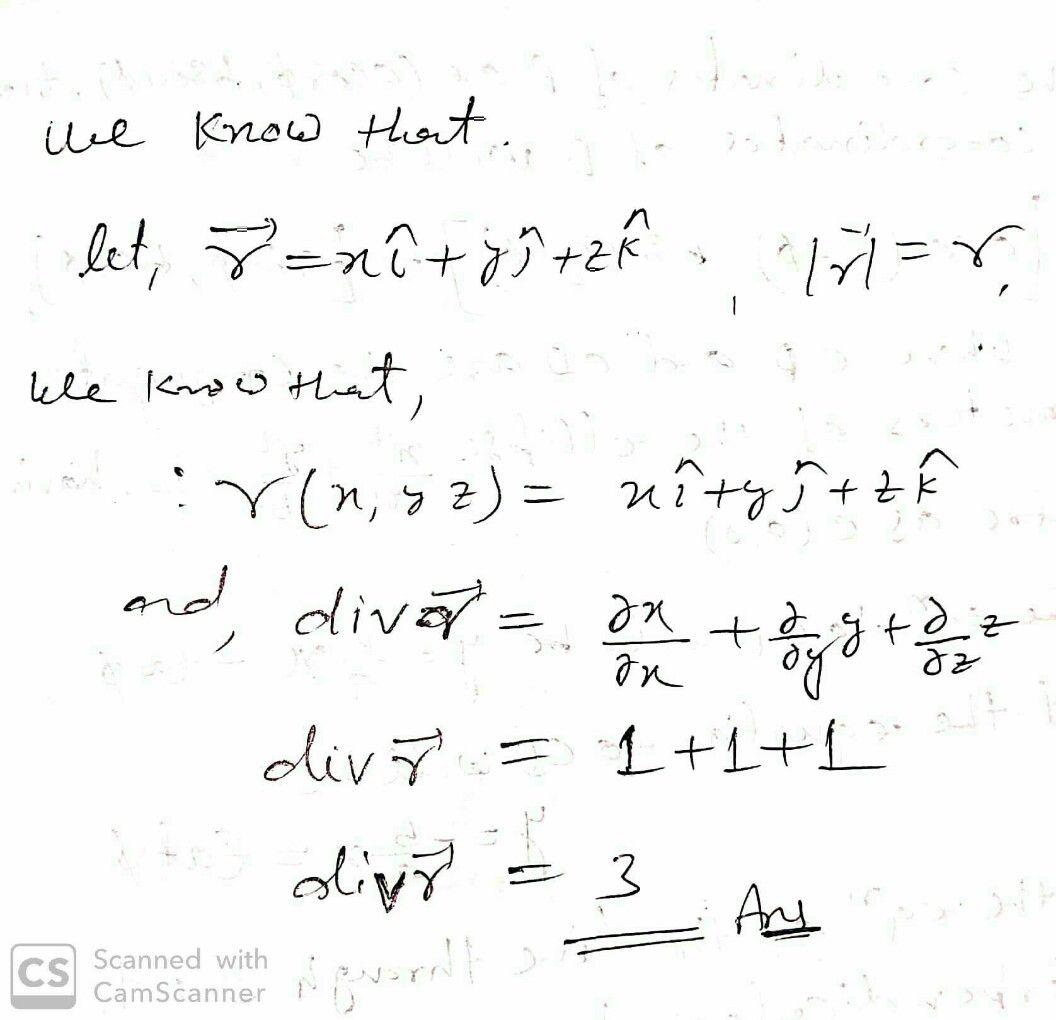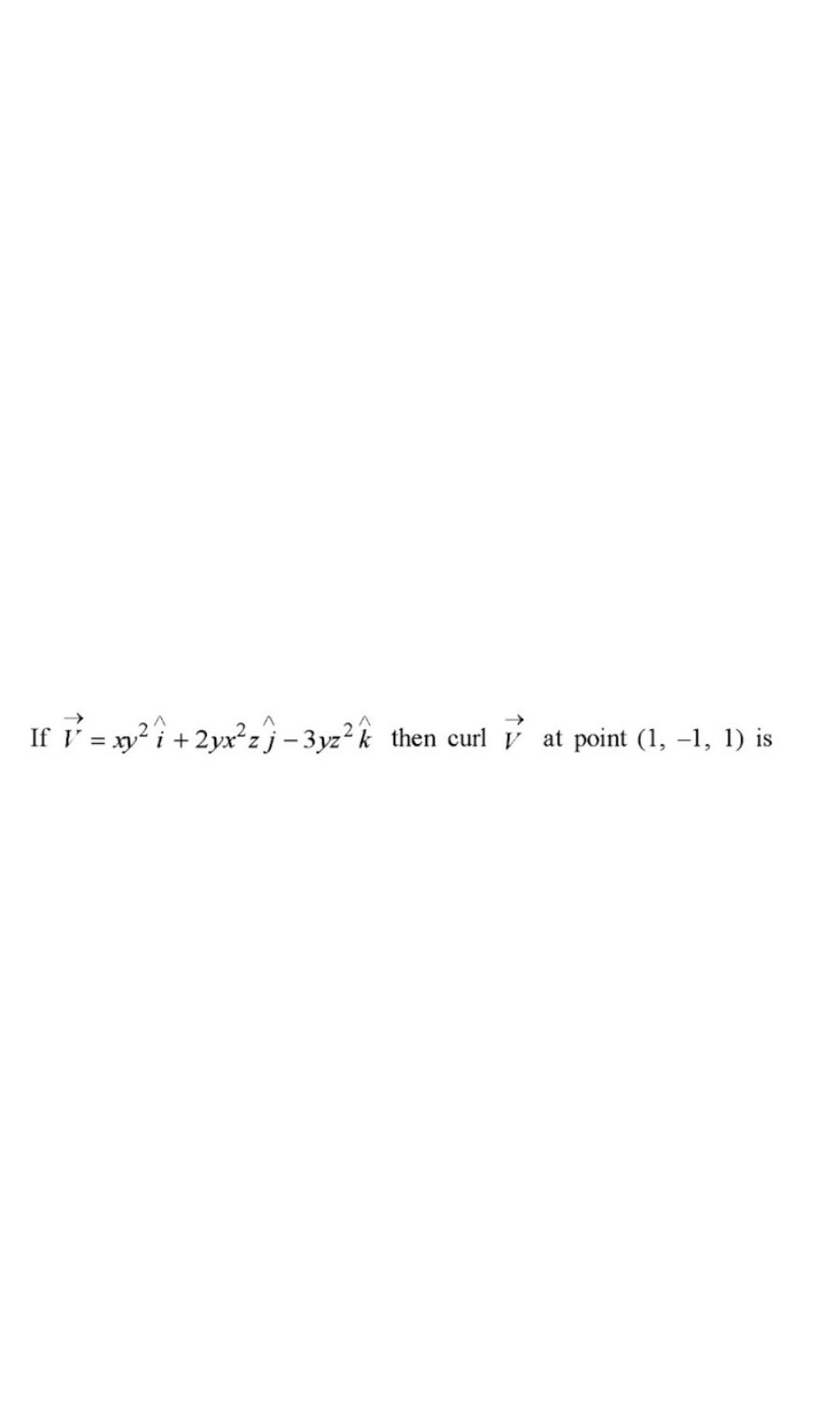Symbol
ProblemIf $\vec{r} =xi+yi+zk$ and $|r1$ $→$ $=r,$ then div $r$
Calculus
Search count: 116
SolutionQanda teacher - tejeswarStudentQanda teacher - tejeswar
Please ask separately
Because it is against the qanda rules
Please be cooperate